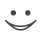# Get these flashcards, study & pass exams. For free! Even on iPhone/Android!

## Converting units (11 Cards)

How to convert inches to feet
We are dividing by the number 12
How do we convert Yards to Feet?
You divide whatever number by Yards then multiply whatever number by Feet.
How do I convert from feet to inches
we are multiplying by 12
How do i convert
Smaller units to the larger units divide

Larger units to smaller units we multiply
How do we go to inches to feet and feet to inches?
We multiply
How do we go feet to yards?
We divide
How do we convert Miles to Feet?
You divide whatever by miles and multiply whatever by feet you will know.
How do we convert cups to ounces?
You divide whatever by cups and multiply whatever by ounces you will know.
How do we convert pints to cups?
Say you divide whatever by pints and multiply by whatever for cups you will know.
How do we convert quarts to pints?
Say you divide whatever by quarts and multiply by whatever for pints you will know.
How do convert gallons to quarts?
Say you divide whatever by gallons and multiply whateverby quarts you will know.Flashcard set info:
Author: hiphopari
Main topic: Mathematics
Topic: Conversions
Published: 05.02.2010
Tags: math

Card tags:
All cards (11)
no tagsReport abuse